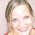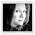### 100.Dear Friends, You have a very dorky blogger on your hands. I have thought of a hundred different ways to post this blog. I'm not joking. This is my 100th post and I've been kind of excited about it. Excited that I have been keeping up with this blog. So in my silliness I had come up with all these ideas of how to present this post... 100 beauty tips, 100 books you should read, 100 places you should visit, etc. I think you get the idea. Instead of all those things I'll discuss my day... pretty normal.

I slept in till 11:00 today. Can you believe it? Mike took care of the kids this morning and I slept and slept. I made eggs, bacon, and toast for brunch. I got to go out and get a cute haircut. I came home and weeded the garden, planted my rose bushes, and used stakes to help my drooping tomato plants.

It's been a relaxing night. I think we're going to get some ice-cream...mmm, good night.

So there it is my 100th post. Nothing grand. Very simple. now done.

Wait, in looking for a fun 100 picture, I stumbled upon the following...

On the Number 100

1) The 50th even number = 2 x 50 = 100
2) The 10th square number = 102 = 10 x 10 = 100
3) Product of the 2nd & 5th square numbers = 22 + 52 = 4 x 25 = 100
4) Product of the 1st prime number & 34th composite numbers = 2 x 50 = 100
5) Product of the 3rd odd & 10th even numbers = 5 x 20 = 100
6) Product of the 5th even & 5th composite numbers = 10 x 10 = 100
7) Sum of the 6th & 8th square numbers = 62 + 82 = 36 + 64 = 100
8) Sum of the 8th prime number & 9th square number = 19 + 81 = 100
9) Sum of the 9th and 10th triangular numbers = 45 + 55 = 100
10) Sum of the 1st four cube numbers 13 + 23 + 33 + 43 = 1 + 8 + 27 + 64 = 100
11) Sum of the 1st ten odd numbers = 1 + 3 + 5 + 7 + 9 + 11 + 13 + 15 + 17 + 19 = 100
12) Sum of the 1st nine prime numbers = 2 + 3 + 5 + 7 + 11 + 13 + 17 + 19 + 23 = 100
13) Log 100 = 2 (logarithm to the base 10)
Ln 100 = 4.60517 (natural logarithm to the base e)
14) The 854th-856th digits of pi = 100
15) The 3036th-3038th digits of phi = 100
16) The boiling point of water = 100o Celsius (centigrade)
17) Pearls are composed of the same substances as oyster shells:
90% calcium carbonate, 5% conchiolin (an organic sceleroprotein), and 5% water.
Molecular weight of Calcium Carbonate =
Ca + C + O3 = 40 + 12 + 3(16) = 40 + 12 + 48 = 100 (100.09)
18) Pythagoreans considered 100 as divinely divine because
it is the square (10 x 10) of the divine decad (10).
19) 100% represents wholeness, purity, or perfection
(no error in a test or no contaminants in a product)Michelle said…
Kinda funny since I just finished watching the movie "23" - an obsession about numbers. But I'm glad you had a relaxing day Jennifer. It sounds like it was lovely.Jenn said…
Fun day friend!